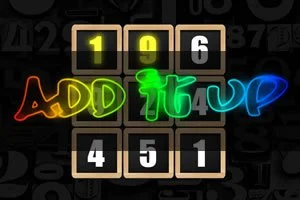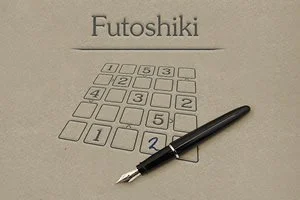# Math games

Play the best free online math games: learn and test your math skills. Learn all about addition, subtraction, multiplication, division, fractions and algebra.### Daily 1 to 25

Daily puzzle game: arrange numbers from 1 to 25.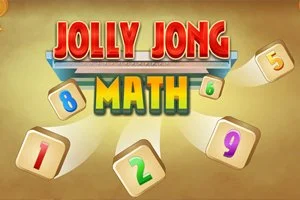### Jolly Jong Math

Combine two tiles and test your math skills.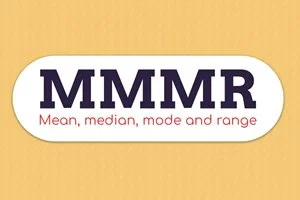### Mean, median, mode and range

Train your math skills in MMMR.### Connect 10

Connect and add up numbers to 10.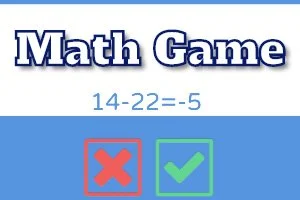### Math Game

Test and train your Math Skills in this game.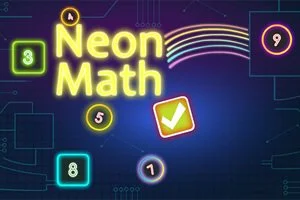### Neon Math

Try to reach 0 on all green numbered tiles.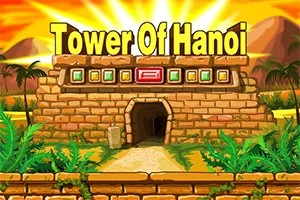### Tower of Hanoi

Classic puzzle game. Move the complete tower.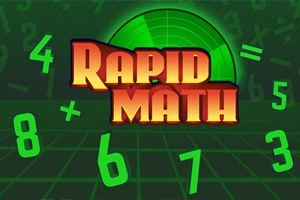### Rapid Math

Solve math operations as fast as possible, gets more difficult as time passes by.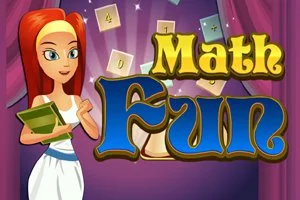### Math Fun

Train your Math Skills in this fun game.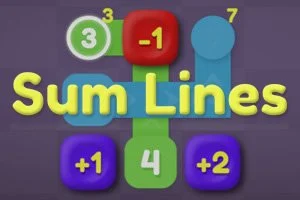### Sum Lines

Drag tiles onto lines and solve the math puzzle.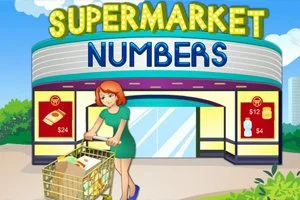### Supermarket Numbers

Test your math skills in this number game.### Daily 15Up

A puzzle game: add up numbers to 15. Solve it with logic.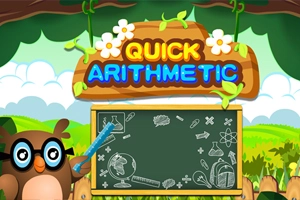### Quick Arithmetic

Test and train your math skills.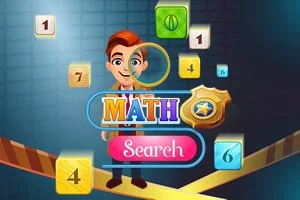### Math Search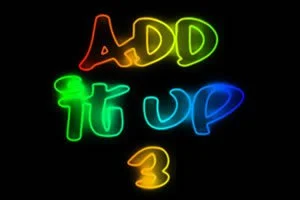Draw a path and add up numbers.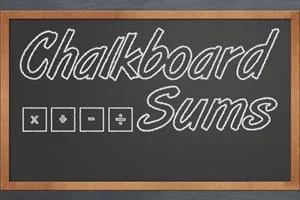### Chalkboard Sums

Brain training: create valid sums with the tiles on the chalkboard.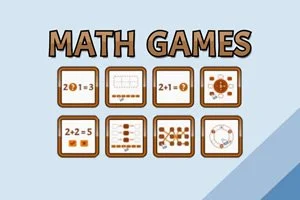### Math Games 8 in 1

Train your Math Skills with 8 mini games.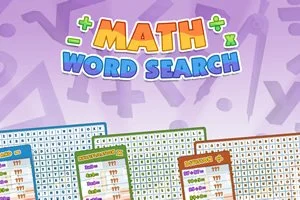### Math Word Search

Train your Math and Word Search skills.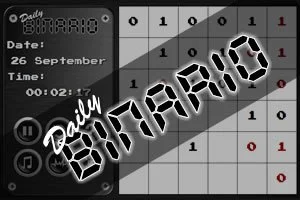### Daily Binario

A puzzle with only 0's and 1's. Solve it with logic.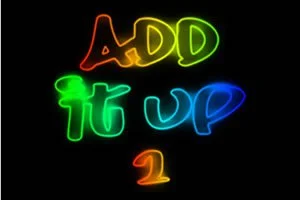Add up the Numbers to a given Target Sum.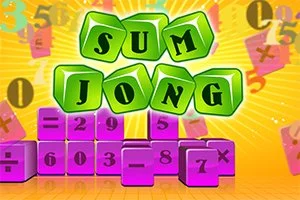### SumJong

Math combined with Mahjong. Test your skills.